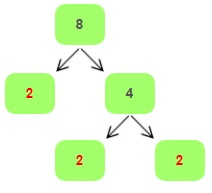## How to Find the Greatest Common Factor (GCF)

Whenever we multiply two whole numbers, we get another number. Now, basically, the numbers we multiplied to get the product, are called the factors of the product. So, for example, $$2 \times 3 = 6$$, this implies that $$2$$ and $$3$$ are the factors of $$6$$. Another conclusion which we can draw from this is, that factors of a number completely divide the number without leaving any remainder.

For ex: Let’s consider the number $$24$$. Now $$24$$ can be divided into factors $$6$$ and $$4$$. Also $$6$$ can be further factorized into $$3$$ and $$2$$. Moreover, $$4$$ can also be factorized into $$2$$ and $$2$$. So, from this we can see that the other factors of $$24$$ are $$3$$, $$8$$ and $$2$$. This is because $$12 \times 2 = 8 \times 3 = 3 \times 8=24$$. Now, let’s learn some facts about factors.

• Each and every number has a smallest factor which is $$1$$.
• Every number has a minimum of two factors that is $$1$$ and the number itself.
• Now, such numbers which have only two factors, i.e., $$1$$ and the number itself are called prime numbers.

#### Prime Factorization

Prime factorization is defined as the product of all the prime factors of a number whose multiplication gives the number itself. Moreover, sometimes to write the prime factors of a number, we may have to repeat the number. So, for example, the factors of $$8$$ are $$1$$ and $$2$$, but to represent $$8$$ we use $$8=2 \times 2 \times 2$$.How to Calculate Prime Factorization of 8?

#### Greatest Common Factor

A common factor is a factor that is common to two numbers. So, as the name suggests, a Greatest Common Factor (GCF) is the greatest numbers formed by multiplying the common factors of 2 numbers. A practical use of Greatest Common Factors is for simplifying fractions.

#### How to Find the Greatest Common Factor (GCF)

To find the Greatest Common Factor, follow these steps:

• First break down both the numbers into their prime factors.
• Now, list out the common factors between those 2 numbers. Then you can multiply the common factors to generate the Greatest Common Factor.
• Also, if you find that there are no common prime factors between these numbers, then the GCF is always 1.

For example, $$100 = 2 \times 2 \times 5 \times 5$$ and $$50 = 2 \times 5 \times 5$$. so $$GCF (100,50) = 2 \times 5 \times 5 = 50$$.

## Find the Greatest Common Factor Video

### Exercises for Greatest Common Factor

1) $$GCF (33, \ 45) \ =$$

2) $$GCF (26, \ 14) \ =$$

3) $$GCF (11, \ 14) \ =$$

4) $$GCF (12, \ 42) \ =$$

5) $$GCF (45, \ 42) \ =$$

6) $$GCF (42, \ 34) \ =$$

7) $$GCF (26, \ 52) \ =$$

8) $$GCF (90, \ 27) \ =$$

9) $$GCF (92, \ 80) \ =$$

10) $$GCF (27, \ 18) \ =$$

1) $$GCF (33, \ 45) \ = \ \color{red}{3}$$
Solution:
Step 1:
List the prime factors of each number:  $$33 = (3, 11)$$  and $$45 = (3, 3, 5)$$
Step 2: The common factor in these two numbers is $$3$$ , therefore their greatest common factor is: $$3$$
WHAT IS THE GREATEST COMMON FACTOR OF 33 AND 45?
2) $$GCF (26, \ 14) \ = \ \color{red}{2}$$
Solution:
Step 1:
List the prime factors of each number:  $$26 = (2, 13)$$  and $$14 = (2, 7 )$$
Step 2: The common factor in these two numbers is $$2$$ , therefore their greatest common factor is: $$2$$
WHAT IS THE GREATEST COMMON FACTOR OF 26 AND 14?
3) $$GCF (11, \ 14) \ = \ \color{red}{1}$$
Solution:
Step 1:
List the prime factors of each number:  $$11 = (11)$$  and $$14 = (2 , 7)$$
Step 2: Because there are no common prime factors, the GCF is $$1$$.
WHAT IS THE GREATEST COMMON FACTOR OF 11 AND 14?
4) $$GCF (12, \ 42) \ = \ \color{red}{6}$$
Solution:
Step 1:
List the prime factors of each number:  $$12 = (2 , 3)$$  and $$42 = (2, 3 , 7)$$
Step 2: The common factors in these two numbers are $$2$$ and $$3$$, therefore their greatest common factor is: $$2 \times 3 =6$$.
WHAT IS THE GREATEST COMMON FACTOR OF 12 AND 42?
5) $$GCF (45, \ 42) \ = \ \color{red}{3}$$ 6) $$GCF (42, \ 34) \ = \ \color{red}{2}$$ 7) $$GCF (26, \ 52) \ = \ \color{red}{26}$$ 8) $$GCF (90, \ 27) \ = \ \color{red}{9}$$ 9) $$GCF (92, \ 80) \ = \ \color{red}{4}$$ 10) $$GCF (27, \ 18) \ = \ \color{red}{9}$$

## Greatest Common Factor Quiz

### ISEE Middle Level Math

$16.99$11.99

### SSAT Middle Level Math Prep 2020

$20.99$15.99

### FTCE Math Study Guide 2020-2021

$20.99$14.99

### AFOQT Math Full Study Guide

$25.99$13.99